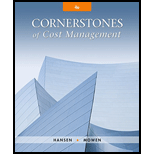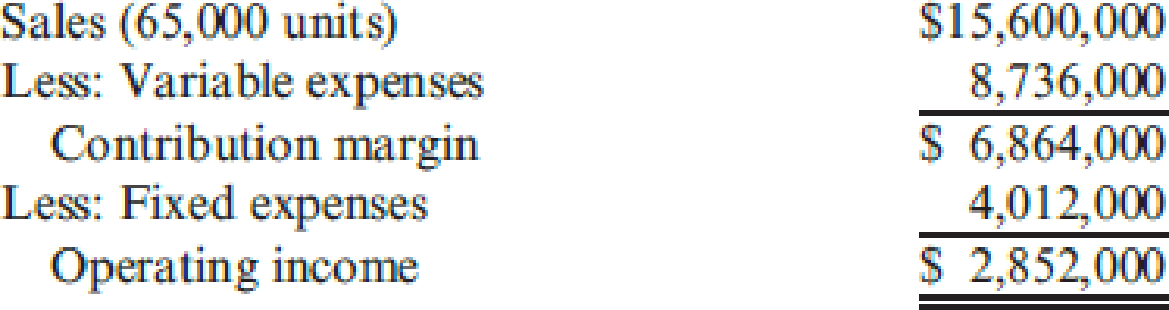# Katayama Company produces a variety of products. One division makes neoprene wetsuits. The division’s projected income statement for the coming year is as follows: Required: 1. Compute the contribution margin per unit, and calculate the break-even point in units. Repeat, using the contribution margin ratio. 2. The divisional manager has decided to increase the advertising budget by $140,000 and cut the average selling price to$200. These actions will increase sales revenues by $1 million. Will this improve the division’s financial situation? Prepare a new income statement to support your answer. 3. Suppose sales revenues exceed the estimated amount on the income statement by$612,000. Without preparing a new income statement, determine by how much profits are underestimated. 4. How many units must be sold to earn an after-tax profit of $1.254 million? Assume a tax rate of 34 percent. (Round your answer up to the next whole unit.) 5. Compute the margin of safety in dollars based on the given income statement. 6. Compute the operating leverage based on the given income statement. (Round to three significant digits.) If sales revenues are 20 percent greater than expected, what is the percentage increase in profits?BuyFindarrow_forward ### Cornerstones of Cost Management (C... 4th Edition Don R. Hansen + 1 other Publisher: Cengage Learning ISBN: 9781305970663 #### Solutions Chapter SectionBuyFindarrow_forward ### Cornerstones of Cost Management (C... 4th Edition Don R. Hansen + 1 other Publisher: Cengage Learning ISBN: 9781305970663 Chapter 16, Problem 37P Textbook Problem 188 views ## Katayama Company produces a variety of products. One division makes neoprene wetsuits. The division’s projected income statement for the coming year is as follows:Required: 1. Compute the contribution margin per unit, and calculate the break-even point in units. Repeat, using the contribution margin ratio. 2. The divisional manager has decided to increase the advertising budget by$140,000 and cut the average selling price to $200. These actions will increase sales revenues by$1 million. Will this improve the division’s financial situation? Prepare a new income statement to support your answer. 3. Suppose sales revenues exceed the estimated amount on the income statement by $612,000. Without preparing a new income statement, determine by how much profits are underestimated. 4. How many units must be sold to earn an after-tax profit of$1.254 million? Assume a tax rate of 34 percent. (Round your answer up to the next whole unit.) 5. Compute the margin of safety in dollars based on the given income statement. 6. Compute the operating leverage based on the given income statement. (Round to three significant digits.) If sales revenues are 20 percent greater than expected, what is the percentage increase in profits?

1.

To determine

Compute the break-even point in units and sales revenue along with the margin of safety.

### Explanation of Solution

Contribution Margin Ratio: The contribution margin ratio shows the amount of difference in the actual sales value and the variable expenses in percentage. This margin indicates that percentage which is available for sale above the fixed costs and the profit. The formula for variable cost ratio is shown below:

Contribution Margin ratio = Contribution MarginSales

Break-Even Point: The break-even point refers to the point of sales at which the firm neither earns a profit nor suffers a loss. It is also known as the point of sales or sales value at which the firm recovers the entire cost of fixed and variable nature.

Compute the contribution margin per unit:

Contribution Margin per unit =Contribution MarginNumber of units=$6,864,00065,000=$105.60

The contribution margin per unit is $105.60. Compute the break-even units: Break-even in Units =Fixed costContribution Margin=$4,012,000$105 2. To determine Compute the income statement showing the financial situation of the firm after the proposed changes. 3. To determine Compute the amount by which the profits are underestimated, if the sales exceed the amount shown in the income statement. 4. To determine Compute the number of units to be sold to generate an after-tax profit of$1.254 million with a tax rate of 34%.

5.

To determine

Compute the margin of safety in sales revenue.

6.

To determine

Compute the operating leverage and the increase in profit percentage if the profit increases.

### Still sussing out bartleby?

Check out a sample textbook solution.

See a sample solution

#### The Solution to Your Study Problems

Bartleby provides explanations to thousands of textbook problems written by our experts, many with advanced degrees!

Get Started

Find more solutions based on key concepts
(Appendix 4B) Explain the difference between the direct method and the sequential method.

Managerial Accounting: The Cornerstone of Business Decision-Making

Organization costs are recorded as an intangible asset and are generally amortized over a period of five years.

College Accounting, Chapters 1-27 (New in Accounting from Heintz and Parry)

Describe the role of prices in market economies.

Principles of Macroeconomics (MindTap Course List)

Describe the four sales channels

Contemporary Marketing

BOND VALUATION Robert Black and Carol Alvarez are vice presidents of Western Money Management and codirectors o...

Fundamentals of Financial Management, Concise Edition (with Thomson ONE - Business School Edition, 1 term (6 months) Printed Access Card) (MindTap Course List)